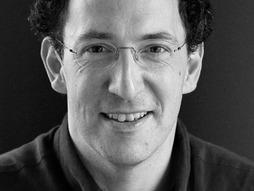Mathematician
Conrad Wolfram runs the worldwide arm of Wolfram Research, the mathematical lab behind the cutting-edge knowledge engine Wolfram Alpha.

### Why you should listen

Conrad Wolfram is the strategic director of Wolfram Research, where his job, in a nutshell, is understanding and finding new uses for the Mathematica technology. Wolfram is especially passionate about finding uses for Mathematica outside of pure computation, using it as a development platform for products that help communicate big ideas. The Demonstrations tool, for instance, makes a compelling case for never writing out another equation -- instead displaying data in interactive, graphical form.

Wolfram's work points up the changing nature of math in the past 30 years, as we've moved from adding machines to calculators to sophisticated math software, allowing us to achieve ever more complex computational feats. But, Wolfram says, many schools are still focused on hand-calculating; using automation, such as a piece of software, to do math is sometimes seen as cheating. This keeps schools from spending the time they need on the new tools of science and mathematics. As they gain significance for everyday living, he suggests, we need to learn to take advantage of these tools and learn to use them young. Learn more at computerbasedmath.org.

### What others say

“What he's saying is that enough time is wasted in math classes on JUST calculation, that often the underlying concept is lost anyway.” — TremorX, YouTube

## More news and ideas from Conrad Wolfram

Education

#### Math class without hand calculation? Estonia is moving toward it

February 13, 2013

Math class should be fascinating, right? At TED2010, Conrad Wolfram suggests that one reason it often isn’t is hand calculation. Most students spend years in math class learning to work sums by hand that a computer can now do. After all, computers are far better at calculation than human beings will ever be, while people […]